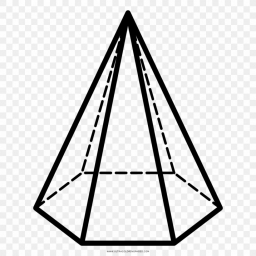# Hexagonal 66574

Find the volume of wax from which the candle in the shape of a regular hexagonal pyramid with a height of 6.5 cm and a length of the base edge of 3 cm is made.

V =  50.6625 cm3

### Step-by-step explanation:Did you find an error or inaccuracy? Feel free to write us. Thank you!

Tips for related online calculators
Need help calculating sum, simplifying, or multiplying fractions? Try our fraction calculator.
The Pythagorean theorem is the base for the right triangle calculator.